# Algorithms Lecture 1: Introduction to Algorithms

23. Sep 2017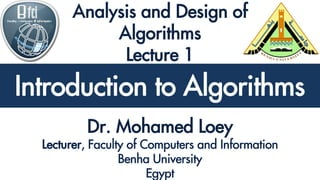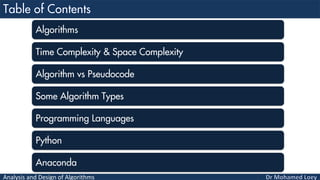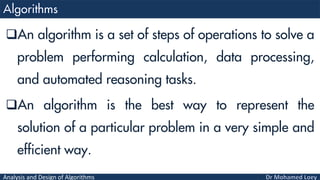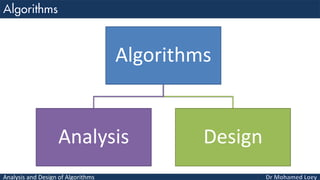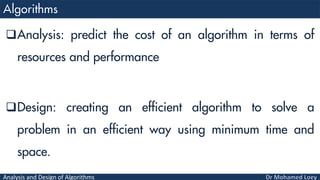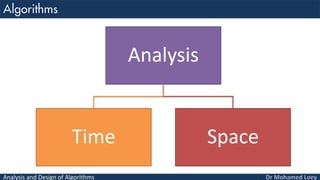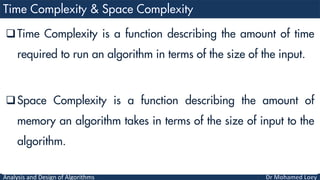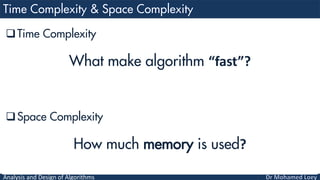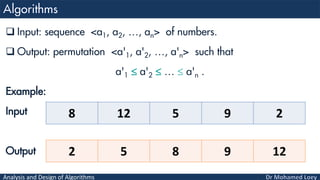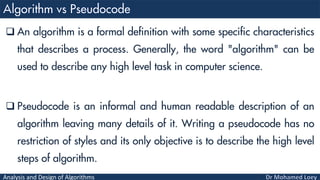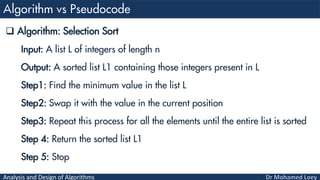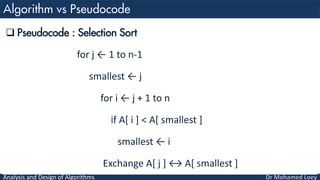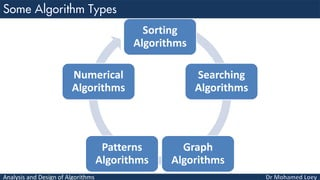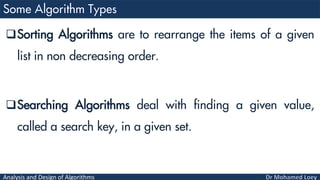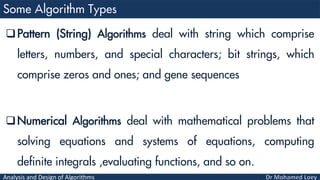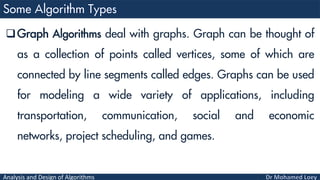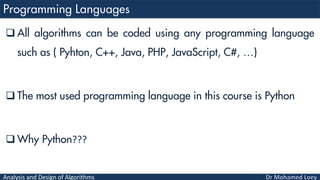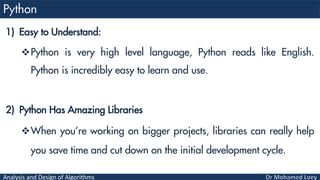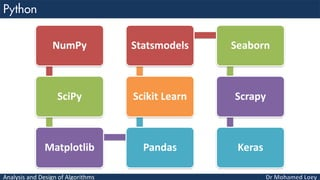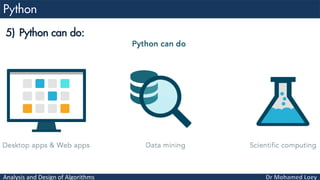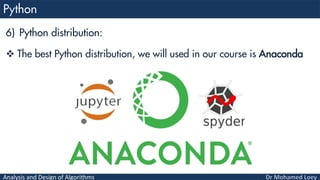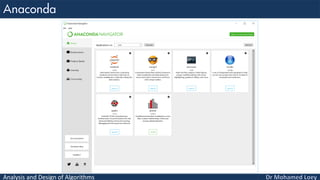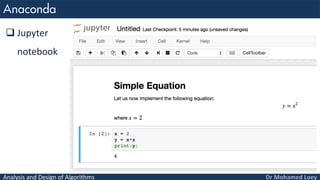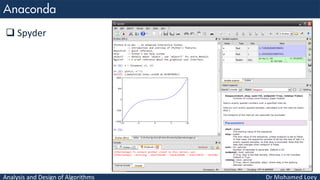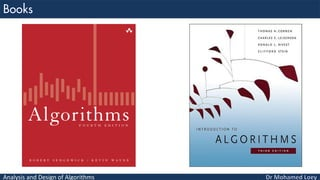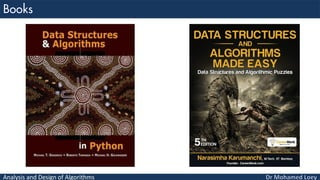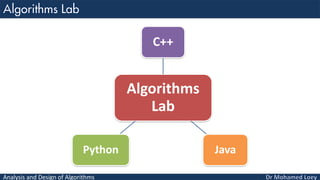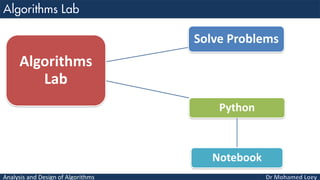1 von 31

### Algorithms Lecture 1: Introduction to Algorithms

• 1. Analysis and Design of Algorithms Introduction to Algorithms
• 2. Analysis and Design of Algorithms Algorithms Time Complexity & Space Complexity Algorithm vs Pseudocode Some Algorithm Types Programming Languages Python Anaconda
• 3. Analysis and Design of Algorithms An algorithm is a set of steps of operations to solve a problem performing calculation, data processing, and automated reasoning tasks. An algorithm is the best way to represent the solution of a particular problem in a very simple and efficient way.
• 4. Analysis and Design of Algorithms Algorithms Analysis Design
• 5. Analysis and Design of Algorithms Analysis: predict the cost of an algorithm in terms of resources and performance Design: creating an efficient algorithm to solve a problem in an efficient way using minimum time and space.
• 6. Analysis and Design of Algorithms Analysis Time Space
• 7. Analysis and Design of Algorithms Time Complexity is a function describing the amount of time required to run an algorithm in terms of the size of the input. Space Complexity is a function describing the amount of memory an algorithm takes in terms of the size of input to the algorithm.
• 8. Analysis and Design of Algorithms Time Complexity What make algorithm “fast”? Space Complexity How much memory is used?
• 9. Analysis and Design of Algorithms  Input: sequence <a1, a2, …, an> of numbers.  Output: permutation <a'1, a'2, …, a'n> such that a'1  a'2  …  a'n . Example: Input Output 8 12 5 9 2 2 5 8 9 12
• 10. Analysis and Design of Algorithms  An algorithm is a formal definition with some specific characteristics that describes a process. Generally, the word "algorithm" can be used to describe any high level task in computer science.  Pseudocode is an informal and human readable description of an algorithm leaving many details of it. Writing a pseudocode has no restriction of styles and its only objective is to describe the high level steps of algorithm.
• 11. Analysis and Design of Algorithms  Algorithm: Selection Sort Input: A list L of integers of length n Output: A sorted list L1 containing those integers present in L Step1: Find the minimum value in the list L Step2: Swap it with the value in the current position Step3: Repeat this process for all the elements until the entire list is sorted Step 4: Return the sorted list L1 Step 5: Stop
• 12. Analysis and Design of Algorithms  Pseudocode : Selection Sort for j ← 1 to n-1 smallest ← j for i ← j + 1 to n if A[ i ] < A[ smallest ] smallest ← i Exchange A[ j ] ↔ A[ smallest ]
• 13. Analysis and Design of Algorithms Sorting Algorithms Searching Algorithms Graph Algorithms Patterns Algorithms Numerical Algorithms
• 14. Analysis and Design of Algorithms Sorting Algorithms are to rearrange the items of a given list in non decreasing order. Searching Algorithms deal with finding a given value, called a search key, in a given set.
• 15. Analysis and Design of Algorithms Pattern (String) Algorithms deal with string which comprise letters, numbers, and special characters; bit strings, which comprise zeros and ones; and gene sequences Numerical Algorithms deal with mathematical problems that solving equations and systems of equations, computing definite integrals ,evaluating functions, and so on.
• 16. Analysis and Design of Algorithms Graph Algorithms deal with graphs. Graph can be thought of as a collection of points called vertices, some of which are connected by line segments called edges. Graphs can be used for modeling a wide variety of applications, including transportation, communication, social and economic networks, project scheduling, and games.
• 17. Analysis and Design of Algorithms  All algorithms can be coded using any programming language such as ( Pyhton, C++, Java, PHP, JavaScript, C#, …)  The most used programming language in this course is Python  Why Python???
• 18. Analysis and Design of Algorithms 1) Easy to Understand: Python is very high level language, Python reads like English. Python is incredibly easy to learn and use. 2) Python Has Amazing Libraries When you’re working on bigger projects, libraries can really help you save time and cut down on the initial development cycle.
• 19. Analysis and Design of Algorithms NumPy SciPy Matplotlib Pandas Scikit Learn Statsmodels Seaborn Scrapy Keras
• 20. Analysis and Design of Algorithms 3) Supportive community Python has documentation, guides, tutorials and more. Plus, the developer community is incredibly active. 4) Great Corporate Sponsor C# has Microsoft, Java has Sun and PHP is used by Facebook. Google adopted Python heavily back in 2006, and they’ve used it for many platforms and applications since.
• 21. Analysis and Design of Algorithms 5) Python can do:
• 22. Analysis and Design of Algorithms 6) Python distribution:  The best Python distribution, we will used in our course is Anaconda
• 23. Analysis and Design of Algorithms
• 24. Analysis and Design of Algorithms  Jupyter notebook
• 25. Analysis and Design of Algorithms  Spyder
• 26. Analysis and Design of Algorithms
• 27. Analysis and Design of Algorithms
• 28. Analysis and Design of Algorithms Algorithms Lab C++ JavaPython
• 29. Analysis and Design of Algorithms Algorithms Lab Solve Problems Python Notebook